# Thermal Conductivity of Metals

Thermal Conductivity is a term analogous to electrical conductivity with a difference that it concerns with the flow of heat unlike current in the case of the latter. It points to the ability of a material to transport heat from one point to another without movement of the material as a whole, the more is the thermal conductivity the better it conducts the heat.
Let us consider a block of material with one end at temperature T1 and other at T2. For T1>T2, heat flows from T1 end to T2 end, and the heat flux(J) flowing across a unit area per unit time is given as-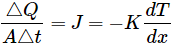Where,
K is the thermal conductivity in Joule/meter-sec-K or Watts/meter-K.

Generally the heat transfer in solid has two components

1. Lattice conduction
2. Electronic conduction

Both types of heat conduction occur in solids but one is dominant over the other depending upon the type of material.
In case of insulating materials, lattice conduction contributes to heat conduction. This is mainly due to the fact that in insulators the electrons are tightly held by their parent atoms and free electrons do not exist. Hence the heat is transferred from one end to another by vibration of atoms held in the lattice structure. Obviously insulators are bad heat conductors since they do not posses enough heat transfer capability due to lack of free electrons.

However in case of metals we have large number of free electrons, and hence heat conduction is primarily due to electronic conduction. The free electrons of the metals can freely move throughout the solid and transfer the thermal energy at a rate very high as compared to insulators. It is due to this that the metals posses high thermal conductivity. It is also observed that among the metals the best electrical conductors also exhibits best thermal conductivity. Since both electrical as well as thermal conductivity is dependent on the free electrons, factors such as alloying effect both the properties.
Thermal conductivity of metals vary from 15 – 450 W/mK at 300K.

## Wiedemann Franz Law

Wiedemann Franz law basically relates the two conductivities of metals, i.e. thermal and electrical conductivity with temperature. It states that the ratio of thermal conductivity K and electrical conductivity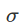is proportional to the temperature of the specimen. G. Wiedemann and R. Franz in 1853 established on the basis of experimental data that the ratio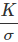is constant at constant temperature.

In 1882 the Danish physicist L. Lorenz demonstrated that the relationchanges in direct proportion to the absolute temperature T.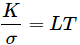Where, T = temperature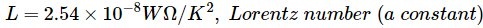This law basically states that with increase in temperature the thermal conductivity of metals increases while the electrical conductivity decreases. We know that the two properties of metals are dependent on the free electrons. An increase in temperature increases the average velocity of the free electrons leading to increase in heat energy transfer. On the other hand increase in the velocity of electrons also increases the number of collisions of the free electrons with lattice ions, and hence contributes to increase in electrical resistivity or reduction in electrical conductivity.
However this law has certain limitations. The proportionality does not holds true for all ranges of temperature. It is only found valid for very high temperatures and very low temperatures. Also certain metals such as beryllium, pure silver etc. do not follow this law.

Want To Learn Faster? 🎓
Get electrical articles delivered to your inbox every week.
No credit card required—it’s 100% free.